Definitions of Square Dance Calls and Concepts
Latch On ({fraction}) [C3A]

Index -->  Plus  |  A1  |  A2  |  C1  |  C2  |  C3A  |  C3B  |  C4  |  NOL  |
Definitions (Text Only) -->  Plus  |  A1  |  A2  |  C1  |  C2  |  C3A  |  C3B  |  C4  |  NOL  |
 Find call:

 \$B8@8l(BFrom Tandem dancers, Facing dancers, or Back-to-Back dancers. EN: 10
Tandem \$B\$N(B 2 \$B?M(B, \$B8~\$+\$\$9g\$C\$?(B 2 \$B?M(B, \$B\$"\$k\$\$\$OGXCf9g\$o\$;\$N(B 2 \$B?M\$+\$i!%(B JP: 10

Right Roll To A Wave, Arm Turn 1/4 (or the designated fraction). EN: 20
Right Roll To A Wave \$B\$r\$7\$F(B, Arm Turn 1/4 (\$B\$"\$k\$\$\$O;X<(\$5\$l\$?(B fraction) \$B\$r\$7\$^\$9!%(B JP: 20

Ends in a R-H Mini-Wave. This is a 2-part call. EN: 30
\$B1&\$N(B Mini-Wave \$B\$G=*\$o\$j(B, 2 \$B%Q!<%H\$N%3!<%k\$G\$9!%(B JP: 30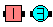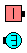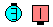Latch On\$B\$NA0(B Right Roll To A Wave\$B\$N8e(B Arm Turn 1/4\$B\$N8e(B (\$B=*\$o\$j(B)

\$B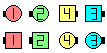\$B\$B;O\$a\$N(B formation: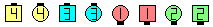As Couples Once Removed Latch On\$B\$N8e(B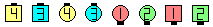Once Removed As Couples Latch On\$B\$N8e(B

1. For the first case, 'As Couples' is heard first, so you work As Couples to do the call 'Once Removed Latch On'. See diagrams below. EN: 40
\$B;O\$a\$N>l9g(B, 'As Couples' \$B\$,:G=i\$KJ9\$3\$(\$k\$N\$G(B, As Couples \$B\$G(B 'Once Removed Latch On' \$B\$N%3!<%k\$r\$7\$^\$9!%JP: 40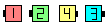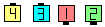Imagine you start herewhere each dancer is a couple EN: 50 1 \$B?M(B 1 \$B?M\$,(B couple \$B\$@\$H;W\$C\$F(B\$B%3!<%k\$r;O\$a\$^\$9(B JP: 50 Once Removed Latch On\$B\$N8e(B
2. For the second case, 'Once Removed' is heard first, so you work Once Removed to do the call 'As Couples Latch On'. Note that, for this case, the dancers in the set {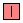,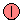,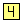,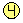} are on every other plane in both the starting formation and the ending formation. Similarly, so are the dancers in the set {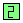,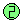,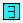,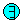}. EN: 60
2 \$B\$DL\\$N>l9g(B, 'Once Removed' \$B\$,:G=i\$KJ9\$3\$(\$k\$N\$G(B, Once Removed \$B\$G(B 'As Couples Latch On' \$B\$N%3!<%k\$r\$7\$J\$1\$l\$P\$J\$j\$^\$;\$s!%\$3\$N>l9g\$KCm0U\$9\$k\$3\$H\$O(B, {,,,} \$B\$N?M\$,(B, \$B;O\$a\$H=*\$o\$j\$NN>J}\$N(B formation \$B\$GF1\$8Cg4V\$G(B, Once Removed \$B\$N0LCV\$K\$\$\$J\$1\$l\$P\$J\$j\$^\$;\$s!%F1MM\$K(B, {,,,} \$B\$N?M\$,F1\$8Cg4V\$G\$9!%(B JP: 60CALLERLAB definition for Latch On (fraction)Choreography for Latch On (fraction)Comments? Questions? Suggestions?

https://www.ceder.net/def/latchon.php?language=japan&action=edit
22-January-2020 16:36:47